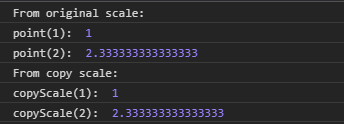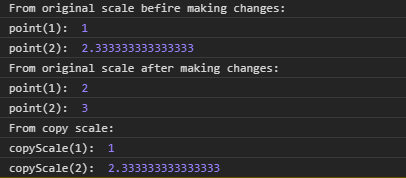# D3.js point.copy() Function

The point.copy() function is used to construct and return the copy of the current scale. Any changes made to either scale is independent of each other i.e change in copy scale will not affect the original scale and vice-versa.

Syntax:

`point.copy();`

Parameters: This function does not accept any parameter.

Return Values: This function returns a copy of the current scale. The return type is a function.

Below given are a few examples of the function given above.

Example 1:

 `  ` `<``html` `lang` `= ``"en"``>  ` `<``head``>  ` `    ``<``meta` `charset` `= ``"UTF-8"` `/>  ` `    ``<``meta` `name` `= ``"viewport"` `        ``path1tent = "``width``=``device``-width,  ` `        ``initial-scale` `= ``1``.0"/>  ` `    ``<``title``>GeekforGeeks  ` `    ``<``script` `src` `= ` `    ``"https://d3js.org/d3.v4.min.js"``> ` `    `` ` `     `  `  ` `<``body``>  ` `    ``<``script``>  ` `// Creating the point scale with specified domain and range. ` `        ``var point = d3.scalePoint() ` `                    ``.domain([1, 2, 3, 4]) ` `                    ``.range([1, 5]); ` `        ``var copyScale = point.copy(); ` `        ``console.log("From original scale: "); ` `        ``console.log("point(1): ", point(1)); ` `        ``console.log("point(2): ", point(2)); ` `        ``console.log("From copy scale: "); ` `        ``console.log("copyScale(1): ", copyScale(1)); ` `        ``console.log("copyScale(2): ", copyScale(2)); ` `         `  `    ``  ` `  ` ` `

Output:Example 2:

 `  ` `<``html` `lang` `= ``"en"``>  ` `<``head``>  ` `    ``<``meta` `charset` `= ``"UTF-8"` `/>  ` `    ``<``meta` `name` `= ``"viewport"` `        ``path1tent = "``width``=``device``-width,  ` `        ``initial-scale` `= ``1``.0"/>  ` `    ``<``title``>GeekforGeeks  ` `    ``<``script` `src` `= ` `    ``"https://d3js.org/d3.v4.min.js"``> ` `    `` ` `     `  `  ` `<``body``>  ` `    ``<``script``>  ` `// Creating the point scale with specified domain and range. ` `        ``var point = d3.scalePoint() ` `                    ``.domain([1, 2, 3, 4]) ` `                    ``.range([1, 5]); ` ` `  `        ``console.log("From original scale befire making changes: "); ` `        ``console.log("point(1): ", point(1)); ` `        ``console.log("point(2): ", point(2)); ` `        ``// Making copy of the original scale ` `        ``var copyScale = point.copy(); ` `        ``point.round([1, 5]); ` `        ``console.log("From original scale after making changes: "); ` `        ``console.log("point(1): ", point(1)); ` `        ``console.log("point(2): ", point(2)); ` ` `  `        ``console.log("From copy scale: "); ` `        ``// Changes in original scale does not ` `        ``// Affect copy scale ` `        ``console.log("copyScale(1): ", copyScale(1)); ` `        ``console.log("copyScale(2): ", copyScale(2)); ` `         `  `    ``  ` `  ` ` `

Output:My Personal Notes arrow_drop_upCheck out this Author's contributed articles.

If you like GeeksforGeeks and would like to contribute, you can also write an article using contribute.geeksforgeeks.org or mail your article to contribute@geeksforgeeks.org. See your article appearing on the GeeksforGeeks main page and help other Geeks.

Please Improve this article if you find anything incorrect by clicking on the "Improve Article" button below.

Article Tags :

Be the First to upvote.

Please write to us at contribute@geeksforgeeks.org to report any issue with the above content.